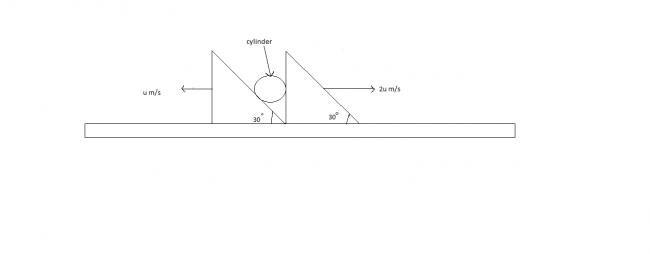# velocityin the given figure find the velocity of the cylinder. assume the cylinder remains in touch with the wedges. all surfaces are frictionless.

•is it √7u

•Aritra Chakrabarti ·

how are you getting it?

•use relative velocity concept.
suppose the left wedge is at rest.
then calculate remaining velocities
finally convert it to velocity w.r.t. ground

•Vivek @ Born this Way ·

Just Exaggerating what Aditya Said :

Sit on the Wedge L (for Left) and Observe: You see that the R (for Right) Wedge is moving away with the velocity ' 3u ' m/s. Now resolving this velocity along the incline and then along the x-y axes, we can get the velocity of the sphere as :

V sa = 3u i - 3√u j

Coming back to the ground frame, Va = - u i

So V sg = V s L + V L

Putting we have V sg = 2u i - √3 u j => | V sg | = √7 u

Notations : sg - Sphere w. r .t ground | l - Wedge L

•Anonymous hey there!!! liked the way of ur thinkin'........................but can u solve it using wedge constraint??
•Aritra Chakrabarti ·

correct.

•